A particle of charge q and mass m is moving along the x-axis with a velocity v and enters a region of electric field E and magnetic field B as shown in the figure below. For which figure the net force on the charge may be zero?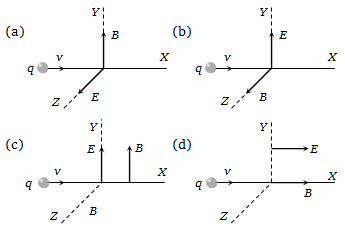Concept Questions :-

Lorentz force
High Yielding Test Series + Question Bank - NEET 2020

Difficulty Level:

A wire carrying a current i is placed in a uniform magnetic field in the form of the curve . The force acting on the wire is: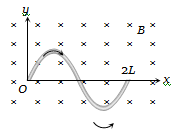1. $\frac{iBL}{\mathrm{\pi }}$                                        2. $iBL\mathrm{\pi }$

3. $2iBL$                                        4. Zero

Concept Questions :-

Lorentz force
High Yielding Test Series + Question Bank - NEET 2020

Difficulty Level:

If induction of magnetic field at a point is B and energy density is U, then which of the following graphs is correct?

1.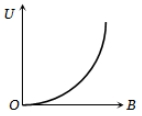2.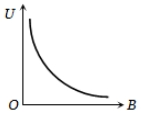3.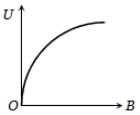4.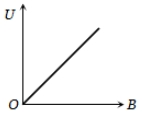Concept Questions :-

Magnetic field due to various cases
High Yielding Test Series + Question Bank - NEET 2020

Difficulty Level:

A long straight wire along the z-axis carries a current I in the negative z-direction. The magnetic field vector $\stackrel{\to }{\mathrm{B}}$ at a point having coordinates (x, y) in the z = 0 plane is :

1. $\frac{{\mathrm{\mu }}_{0}\mathrm{I}\left(\mathrm{y}\stackrel{^}{\mathrm{i}}-\mathrm{x}\stackrel{^}{\mathrm{j}}\right)}{2\mathrm{\pi }\left({\mathrm{x}}^{2}+{\mathrm{y}}^{2}\right)}$

2. $\frac{{\mathrm{\mu }}_{0}\mathrm{I}\left(\mathrm{x}\stackrel{^}{\mathrm{i}}+\mathrm{y}\stackrel{^}{\mathrm{j}}\right)}{2\mathrm{\pi }\left({\mathrm{x}}^{2}+{\mathrm{y}}^{2}\right)}$

3. $\frac{{\mathrm{\mu }}_{0}\mathrm{I}\left(\mathrm{x}\stackrel{^}{\mathrm{j}}-\mathrm{y}\stackrel{^}{\mathrm{i}}\right)}{2\mathrm{\pi }\left({\mathrm{x}}^{2}+{\mathrm{y}}^{2}\right)}$

4. $\frac{{\mathrm{\mu }}_{0}\mathrm{I}\left(\mathrm{x}\stackrel{^}{\mathrm{i}}-\mathrm{y}\stackrel{^}{\mathrm{j}}\right)}{2\mathrm{\pi }\left({\mathrm{x}}^{2}+{\mathrm{y}}^{2}\right)}$

Concept Questions :-

Magnetic field due to various cases
High Yielding Test Series + Question Bank - NEET 2020

Difficulty Level:

A circular coil is in y-z plane with centre at origin. The coil is carrying a constant current. Assuming direction of magnetic field at x = – 25 cm to be positive direction of magnetic field, which of the following graphs shows variation of magnetic field along x-axis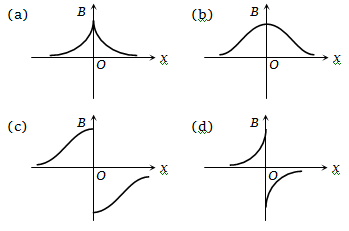Concept Questions :-

Magnetic field due to various cases
High Yielding Test Series + Question Bank - NEET 2020

Difficulty Level:

The ratio of the magnetic field at the centre of a current carrying circular wire and the magnetic field at the centre of a square coil made from the same length of wire will be

(a) $\frac{{\mathrm{\pi }}^{2}}{4\sqrt{2}}$                             (b) $\frac{{\mathrm{\pi }}^{2}}{8\sqrt{2}}$

(c) $\frac{\mathrm{\pi }}{2\sqrt{2}}$                             (d) $\frac{\mathrm{\pi }}{4\sqrt{2}}$

Concept Questions :-

Magnetic field due to various cases
High Yielding Test Series + Question Bank - NEET 2020

Difficulty Level:

A particle of charge +q and mass m moving under the influence of a uniform electric field $\mathrm{E}\stackrel{^}{\mathrm{i}}$ and a uniform magnetic field $\mathrm{B}\stackrel{^}{\mathrm{k}}$ follows trajectory from P to Q as shown in figure. The velocities at P and Q are $\mathrm{v}\stackrel{^}{\mathrm{i}}$ and $-2\mathrm{v}\stackrel{^}{\mathrm{j}}$ respectively. Which of the following statement(s) is/are correct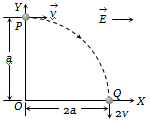(a) $\mathrm{E}=\frac{3}{4}\frac{{\mathrm{mv}}^{2}}{\mathrm{qa}}$

(b) Rate of work done by electric field at P is $\frac{3}{4}\frac{{\mathrm{mv}}^{3}}{\mathrm{a}}$

(c)  Rate of  work done by both fields at Q is zero

(d) All of the above

Concept Questions :-

Lorentz force
High Yielding Test Series + Question Bank - NEET 2020

Difficulty Level:

Figure shows a square loop ABCD with edge length a. The resistance of the wire ABC is r and that of ADC is 2r. The value of magnetic field at the centre of the loop assuming uniform wire is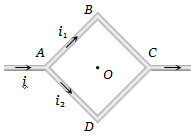(a) $\frac{\sqrt{2}{\mu }_{0}i}{3\mathrm{\pi a}}\odot$                                  (b) $\frac{\sqrt{2}{\mu }_{0}i}{3\mathrm{\pi a}}\otimes$

(c) $\frac{\sqrt{2}{\mu }_{0}i}{\mathrm{\pi a}}\odot$                                   (d) $\frac{\sqrt{2}{\mu }_{0}i}{\mathrm{\pi a}}\otimes$

Concept Questions :-

Magnetic field due to various cases
High Yielding Test Series + Question Bank - NEET 2020

Difficulty Level:

For a positively charged particle moving in a  x- plane initially along the x-axis, there is a sudden change in its path due to the presence of electric and/or magnetic fields beyond P. The curved path is shown in the x-y plane and is found to be non-circular. Which one of the following combinations is possible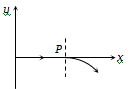(a)                   (b)

(c) $\stackrel{\to }{\mathrm{E}}=0;\stackrel{\to }{\mathrm{B}}=\mathrm{c}\stackrel{^}{\mathrm{j}}+\mathrm{b}\stackrel{^}{\mathrm{k}}$                    (d)  $\stackrel{\to }{\mathrm{E}}=\mathrm{ai};\stackrel{\to }{\mathrm{B}}=\mathrm{c}\stackrel{^}{\mathrm{k}}+\mathrm{b}\stackrel{^}{\mathrm{j}}$

Concept Questions :-

Lorentz force
High Yielding Test Series + Question Bank - NEET 2020

Difficulty Level:

Figure shows the cross-sectional view of the partially hollow cylindrical conductor with inner radius 'R' and outer radius '2R' carrying uniformly distributed current along it's axis. The magnetic induction at point 'P' at a distance $\frac{3R}{2}$ from the axis of the cylinder will be: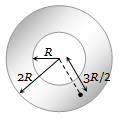1. Zero

2. $\frac{5{\mu }_{0}i}{72\mathrm{\pi R}}$

3. $\frac{7{\mu }_{0}i}{18\mathrm{\pi R}}$

4.  $\frac{5{\mu }_{o}i}{36\mathrm{\pi R}}$

Concept Questions :-

Magnetic field due to various cases